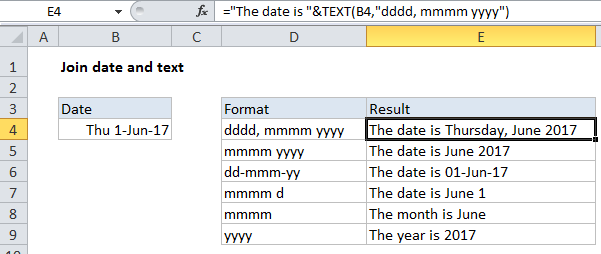## Excel Office

Excel How Tos, Tutorials, Tips & Tricks, Shortcuts

# How to join date and text together in Excel

To join a date with text, you can use concatenation with the TEXT function to control the date format.Generic formula

`="text"&TEXT(date,format)`Note: To control the date format, we use the TEXT function, which is designed to convert a number into text using a specified number format.

## Explanation

In the example shown, the formula in E4 is:

`="The date is "&TEXT(B4,"dddd, mmmm yyyy")`

### How this formula works

The core of this formula is concatenation using the ampersand (&) operator:

`="The date is"&date`

However, if you simply join a date with text and don’t control the date format, the date will revert to it’s raw serial number format:

Also See:   Pad week numbers with zeros in Excel

The date is 42887